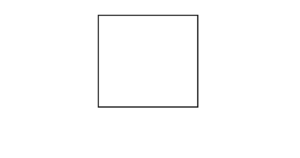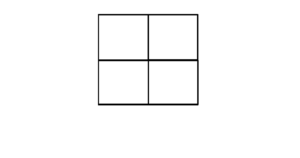# Find the number of squares inside the given square grid

Given a grid of side N * N, the task is to find the total number of squares that exist inside it. All squares selected can be of any length.

Examples:

Input: N = 1
Output: 1Input: N = 2
Output: 5Input: N = 4
Output: 30

## Recommended: Please try your approach on {IDE} first, before moving on to the solution.

Approach: Taking a few examples, it can be observed that for a grid on size N * N, the number of squares inside it will be 12 + 22 + 32 + … + N2

Below is the implementation of the above approach:

## C++

 `// C++ implementation of the approach ` `#include ` `using` `namespace` `std; ` ` `  `// Function to return the number ` `// of squares inside an n*n grid ` `int` `cntSquares(``int` `n) ` `{ ` `    ``int` `squares = 0; ` `    ``for` `(``int` `i = 1; i <= n; i++) { ` `        ``squares += ``pow``(i, 2); ` `    ``} ` `    ``return` `squares; ` `} ` ` `  `// Driver code ` `int` `main() ` `{ ` `    ``int` `n = 4; ` ` `  `    ``cout << cntSquares(4); ` ` `  `    ``return` `0; ` `} `

## Java

 `// Java implementation of the approach ` `class` `GFG { ` ` `  `    ``// Function to return the number ` `    ``// of squares inside an n*n grid ` `    ``static` `int` `cntSquares(``int` `n) ` `    ``{ ` `        ``int` `squares = ``0``; ` `        ``for` `(``int` `i = ``1``; i <= n; i++) { ` `            ``squares += Math.pow(i, ``2``); ` `        ``} ` `        ``return` `squares; ` `    ``} ` ` `  `    ``// Driver code ` `    ``public` `static` `void` `main(String args[]) ` `    ``{ ` `        ``int` `n = ``4``; ` ` `  `        ``System.out.print(cntSquares(``4``)); ` `    ``} ` `} `

## Python3

 `# Python3 implementation of the approach  ` ` `  `# Function to return the number  ` `# of squares inside an n*n grid  ` `def` `cntSquares(n) :  ` ` `  `    ``squares ``=` `0``;  ` `    ``for` `i ``in` `range``(``1``, n ``+` `1``) : ` `        ``squares ``+``=` `i ``*``*` `2``;  ` ` `  `    ``return` `squares;  ` ` `  `# Driver code  ` `if` `__name__ ``=``=` `"__main__"` `:  ` ` `  `    ``n ``=` `4``;  ` ` `  `    ``print``(cntSquares(``4``));  ` ` `  `# This code is contributed by AnkitRai01 `

## C#

 `// C# implementation of the approach ` `using` `System; ` ` `  `class` `GFG  ` `{ ` ` `  `    ``// Function to return the number ` `    ``// of squares inside an n*n grid ` `    ``static` `int` `cntSquares(``int` `n) ` `    ``{ ` `        ``int` `squares = 0; ` `        ``for` `(``int` `i = 1; i <= n; i++)  ` `        ``{ ` `            ``squares += (``int``)Math.Pow(i, 2); ` `        ``} ` `        ``return` `squares; ` `    ``} ` ` `  `    ``// Driver code ` `    ``public` `static` `void` `Main(String []args) ` `    ``{ ` `        ``int` `n = 4; ` ` `  `        ``Console.Write(cntSquares(n)); ` `    ``} ` `} ` ` `  `// This code is contributed by 29AjayKumar `

Output:

```30
```

My Personal Notes arrow_drop_upCheck out this Author's contributed articles.

If you like GeeksforGeeks and would like to contribute, you can also write an article using contribute.geeksforgeeks.org or mail your article to contribute@geeksforgeeks.org. See your article appearing on the GeeksforGeeks main page and help other Geeks.

Please Improve this article if you find anything incorrect by clicking on the "Improve Article" button below.

Improved By : 29AjayKumar, AnkitRai01

Article Tags :
Practice Tags :

1

Please write to us at contribute@geeksforgeeks.org to report any issue with the above content.